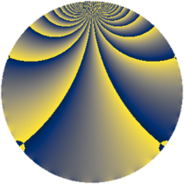# Properties

 Label 1840.2.rLevel $1840$ Weight $2$ Character orbit 1840.r Rep. character $\chi_{1840}(413,\cdot)$ Character field $\Q(\zeta_{4})$ Dimension $568$ Sturm bound $576$

# Related objects

## Defining parameters

 Level: $$N$$ $$=$$ $$1840 = 2^{4} \cdot 5 \cdot 23$$ Weight: $$k$$ $$=$$ $$2$$ Character orbit: $$[\chi]$$ $$=$$ 1840.r (of order $$4$$ and degree $$2$$) Character conductor: $$\operatorname{cond}(\chi)$$ $$=$$ $$1840$$ Character field: $$\Q(i)$$ Sturm bound: $$576$$

## Dimensions

The following table gives the dimensions of various subspaces of $$M_{2}(1840, [\chi])$$.

Total New Old
Modular forms 584 584 0
Cusp forms 568 568 0
Eisenstein series 16 16 0

## Trace form

 $$568q - 4q^{2} - 8q^{4} - 8q^{6} - 4q^{8} - 552q^{9} + O(q^{10})$$ $$568q - 4q^{2} - 8q^{4} - 8q^{6} - 4q^{8} - 552q^{9} + 8q^{12} - 16q^{16} + 24q^{24} - 8q^{26} - 16q^{31} - 24q^{32} - 24q^{35} - 8q^{36} + 48q^{39} - 20q^{46} - 8q^{47} - 76q^{48} - 24q^{50} + 8q^{52} + 40q^{54} + 20q^{58} + 44q^{62} - 32q^{64} - 4q^{69} - 16q^{70} - 24q^{72} + 8q^{75} - 64q^{77} - 28q^{78} + 488q^{81} + 4q^{82} - 24q^{85} + 24q^{87} - 76q^{92} - 8q^{94} - 8q^{95} - 8q^{96} + 60q^{98} + O(q^{100})$$

## Decomposition of $$S_{2}^{\mathrm{new}}(1840, [\chi])$$ into newform subspaces

The newforms in this space have not yet been added to the LMFDB.